# Euler's Formula and Topology

##### Age 16 to 18

Published 2000 Revised 2009

## Euler's formula for the sphere

Roughly speaking, a network (or, as mathematicians would say, a graph ) is a collection of points, called vertices , and lines joining them, called edges . Each edge meets only two vertices (one at each of its ends), and two edges must not intersect except at a vertex (which will then be a common endpoint of the two edges). The collection of edges form the boundary of certain areas, called faces . Faces must not have holes in them or handles on them. If two faces have common boundary points, then they must share a common edge (and only this), or a common vertex (and only this).

In Figure 1 there are two examples of networks, and these have
3 faces, 12 edges and 10 vertices,
and
(ii) 2 faces, 6 edges and 5 vertices,
respectively.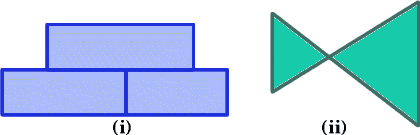Figure 1

It is not necessary to assume that the lines in a network are straight lines'; they can be curves' of any sort except that they must not cross over themselves or each other. Moreover, we can draw such networks on any surface (for example, the plane, a sphere, a cylinder, and so on).

A triangulation of a surface is a network on the surface all of whose faces are triangular (that is, they are bounded by three edges). In fact, surveyors map the countryside by triangulating it with trig points' (usually on mountain tops) and measuring angles and distances between these trig points. In this case the trig points are the vertices of the triangulation.

The simplest example of a triangulation on the sphere (which we think of as the surface of the earth) is found by drawing the equator and, say, $n$ lines of longitude. In this example, which is illustrated in Figure 2, there are $2n$ triangular faces, $n+2$ vertices ($n$ on the equator, and one at each pole), and $3n$ edges, so that $$\hbox{(number of faces)}-\hbox{(number of edges)}+ \hbox{(number of vertices)} = 2n - 3n + (n + 2) = 2.$$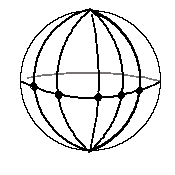Figure 2

It is perhaps surprising that this answer does not depend on the choice of $n$ . Even more remarkable is the fact, known as Euler's Theorem , that this formula holds for ALL triangulations of the sphere. Leonard Euler (1707-1783) was a Swiss mathematician who was, perhaps, the most productive mathematician of all time. Thus, for any triangulation of the sphere with, say, $T$ triangles, $E$ edges and $V$ vertices, Euler's formula for the sphere is that $$T-E+V = 2.$$

The important thing to realise is that this formula is a topological invariant : this means that if we deform the triangulation and the sphere continuously then the numbers $T$, $E$ and $V$ will not change and the formula will still be true. For example, as we can deform the sphere to a cube, the formula will still hold for a cube, so let us check this with one particular triangulation of the cube. We divide each face of the cube into two triangles by drawing a diagonal across each face. Then there are six faces of the cube and each gives two triangles; thus $T=12$. Clearly $V=8$ (there are no new vertices introduced when we draw the diagonals), and $E = 18$ (twelve from the original cube and the six added diagonals). Thus $$T-E+V = 12-18+8 = 2.$$

### EXERCISE 1

Check that Euler's formula is true in each of the following cases (and in each case you should try different triangulations):
1. for a tetrahedron (a pyramid with a triangular base);
2. for the surface formed by gluing two tetrahedra together across their base;
3. for a pyramid with a square base as found in Egypt (remember that the base of the pyramid, where the pyramid touches the ground, is part of the surface of the pyramid);
4. for the octahedron (which is formed by gluing two pyramids together across their base);
5. the surface formed by gluing two pyramids, each with a square base, onto two different faces of a cube. You should assume that the faces of the cube are the same size as the bases of the pyramids.

### EXERCISE 2

Construct your own surface (for example, by sticking several cubes together), triangulate it, and then check that Euler's formula works for this.

#### Remark 1:

Construct' here does not mean that you should physically construct the surface; part of mathematics is learning how to represent three dimensional objects on a sheet of paper!

#### Remark:

the surface must be a deformed sphere; for example, Euler's formula as given above will not hold on the surface of a doughnut (a torus).

### Legendre's proof of Euler's formula on a sphere

The geometry of the sphere is extremely important; for example, when navigators (in ships or planes) work out their course across one of the oceans they must use the geometry of the sphere (and not the geometry of the plane!). In the usual plane Euclidean geometry, the angle sum of a triangle is $180$ degrees (or $\pi$ radians). On the surface of the sphere, the arc of shortest distance between two points is the arc of a great circle on the sphere, and if we form a spherical' triangle with these arcs as sides then the angle sum of the triangle is NOT $180$ degrees. For example, if we look at the triangle on the sphere bounded by the equator, the Greenwich meridian, and the line of longitude given by $90^\circ$ East, we obtain a spherical triangle all three of whose angles are $90^\circ$. Let us call this triangle $\Delta$. You may like to consider now what other angle sums of a triangle are possible on the sphere.

It is a fact, which we shall assume here, that if a spherical triangle is lying on a sphere of unit radius and has, say, angles $\theta_1$, $\theta_2$ and $\theta_3$ (measured in radians), then the area of the triangle is $\theta_1+\theta_2+\theta_3 - \pi$. Of course, in Euclidean geometry, we would have $\theta_1+\theta_2+\theta_3 = \pi$, but this formula is no longer valid because our space is curved. At its simplest level, Einstein's theory about space-time is that the space-time we live in is curved and so the formulae' of physics are different to those that we get if we just use the Euclidean point of view.

Notice that if we accept the above formula for the area of a spherical triangle, then the triangle $\Delta$ constructed above has area $3(\pi/2)-\pi = \pi/2$. As eight copies of $\Delta$ will fill the sphere without overlapping, we see that the area of the sphere (of unit radius) is $4\pi$.

We can now give Legendre's beautiful proof of Euler's formula that is based on a simple discussion of geometry on the sphere. For any triangle on the sphere, we have $$\hbox{angle sum of triangle } = \hbox{area of triangle} + \pi.$$ Suppose that there are $T$ triangles, $E$ edges and $V$ vertices in the triangulation of the sphere. Then, summing all angles in all triangles, the total angle sum is $2\pi V$ (as all of the angles occur at a vertex without overlap, and the angle sum at any one of the $V$ vertices is exactly $2\pi$). Also, the sum of the areas of the triangles is the area of the sphere, namely $4\pi$; thus we see that $$2\pi V = 4\pi + T\pi ,$$ or $$V - T/2 = 2.$$ Now (by counting edges of each triangle and noting that this counts each edge twice), we obtain $3T=2E$, or $E=3T/2$. Thus we have $$T - E + V = T - 3T/2 + (2 + T/2)= 2$$ which is Euler's formula.

### Euler's formula for a simple closed polygon

Given a polygon that does not cross itself, we can triangulate the inside of the polygon into non-overlapping triangles such that any two triangles meet (if at all) either along a common edge, or at a common vertex. Suppose that there are $T$ triangles, $E$ edges and $V$ vertices; then Euler's formula for a polygon is $$T-E+V = 1.$$ You should be able to confirm this in the polygon illustrated in Figure 3.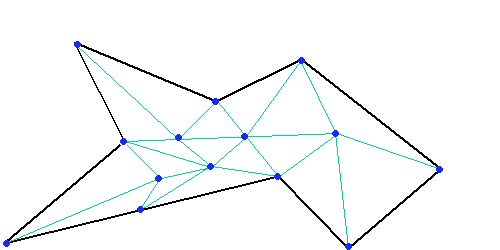Figure 3

### EXERCISE 3

Draw different polygons, triangulate them, and check that Euler's formula is true.

### Proof of Euler's formula for a simple closed polygon

.

Because the terms $T$, $E$ and $V$ are unchanged when we apply a continuous change (or deformation) to the picture, we can imagine the picture to be drawn on a flexible rubber sheet. We cut the polygon out of the sheet, and then manipulate it until it fits exactly onto the lower half of a sphere sitting on the table. We can now regard it as a triangulation of the lower hemisphere of this sphere, and we shall use geographical terms, like equator' and north pole' to describe the points on the sphere. There will be a certain number of vertices, say $N$, on the equator' of the sphere (exactly the same number as were on the boundary of the original polygon) and, as the vertices and edges alternate around the equator, there will also be exactly $N$ edges on the equator. Now draw arcs of circles from the North pole' of the sphere to each of these $N$ vertices (see Figure 4). This will now give us a triangulation of the sphere with $N$ new triangular faces, $N$ new edges (all from the North pole) and one new vertex (at the North pole). Thus, from Euler's formula for the sphere, $$(T+N) - (E+N) + (V+1) = 2,$$ and this gives Euler's formula for the polygon, namely $$T-E+V = 1.$$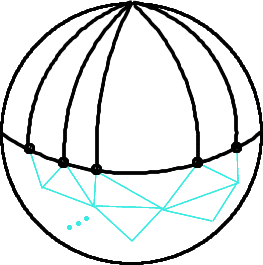Figure 4

### Euler's formula with polygonal faces on any surface

So far we have considered Euler's formula on a surface with the network only having triangular faces. In fact, the formula also holds when the faces are polygons. You should try some examples of this to persuade yourself that this is so. As one example, the cube has six square faces, 12 edges and 8 vertices and 6 - 12 + 8 = 2.

Here is a sketch of a proof of Euler's formula for a sphere $$F - E + V = 2.$$ Some details are omitted but the general idea should be clear.

We start with a polygon drawn on the sphere. Suppose this has $N$ vertices and hence $N$ sides on the boundary of the polygon. There are two faces (the inside and outside of the polygon) so for this network we have $$F - E + V = 2 - N + N = 2.$$ Suppose we now join two vertices by a polygonal curve - shown as a red line in the diagram.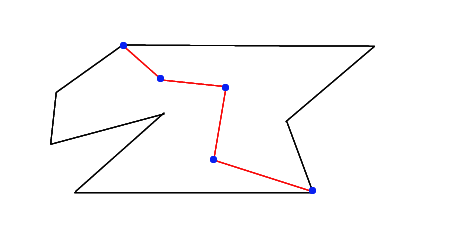For a general curve of this type we add $k$ new edges, $k - 1$ new vertices (in the figure, $k = 4$), and the number of faces is increased by one (one face is divided into two). Then for the new network $$F_{new} - E_{new} + V_{new} = (F + 1) - (E + k) + (V + k - 1)= F - E + V = 2.$$ In the same way we can add another 'dotted' line without changing $F - E + V$, and in this way we can build a general network for which we must still have $F - E + V = 2.$

For a polygon the proof is very much like the proof in Section 3.

### A Euler's formula for a polygon with holes

Consider two polygons, one inside the other; some examples are given in Figure 5. What is Euler's formula for the region between the two polygons ? You should draw different regions of this type, triangulate them in different ways, and when you have reached an answer experimentally, try and prove it.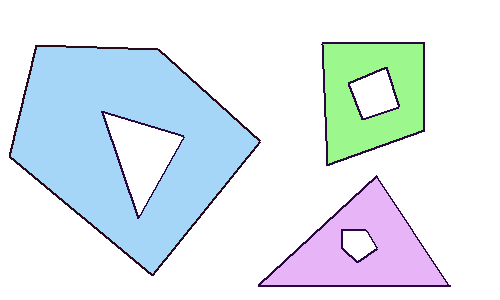Figure 5

Now try to find Euler's formula for regions with two or more holes like those in Figure 6.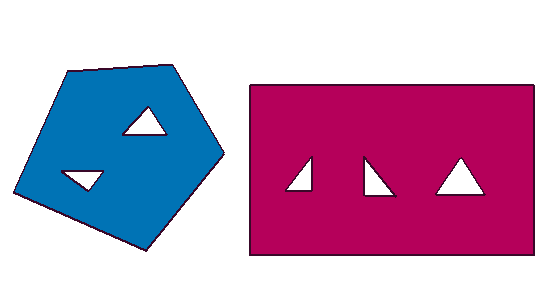Figure 6

### A Euler's formula for a square tube

Consider a square tube with no ends as illustrated in Figure 7, and consider triangulations of this that cover the whole tube. What is Euler's formula for a square tube ? You should draw different triangulations of this, and when you have reached an answer experimentally, try and prove it.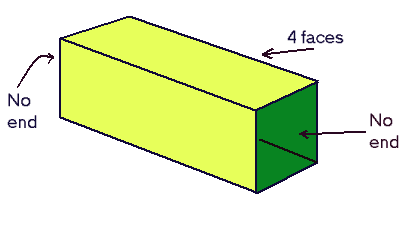Figure 7

When you have done this, try and find Euler's formula for a doughnut' : see Figure 8.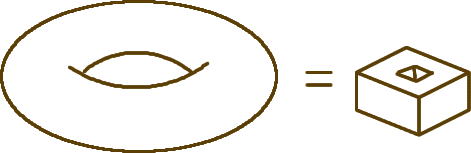Figure 8

### The angle deficiency of a polyhedron

Here is an attractive application of Euler's Formula. The angle deficiency of a vertex of a polyhedron is $360^\circ$ (or $2\pi$ radians) minus the sum of the angles at the vertex of the faces that meet at the vertex. For example, for a cube, there are three faces, each with a right angle at each vertex so the angle deficiency (at each vertex) is $2\pi - 3\times (\pi/2)\ = \pi /2$ ($360^\circ \ - 3\times90^\circ = 90^\circ$), or 90 degrees. The total angle deficiency for the cube is $8\times 90^\circ\ = 720^\circ$ (or $4\pi$ radians). It is a remarkable fact that the total angle deficiency of any polyhedron that is topologically equivalent to a sphere is $720^\circ$ or $4\pi$ radians .

Suppose that we have a polyhedron which is made up with faces $F_1,\ldots F_k$ (each of these is a polygon, which need not be regular). Then we can apply Euler's Theorem to the polyhedron, so let us count the faces, edges and vertices. First, by definition, there are $k$ faces. Suppose that the face $F_j$ has $N_j$ edges (and hence $N_j$ vertices). If we count the total number of edges by looking at them from inside each face we see' $N_1+\cdots +N_k$ edges. But of course, we see each edge twice (for each edge is seen' from both sides); thus $$2E = N_1+\cdots + N_k.$$ One interesting fact here is that $N_1+\cdots +N_k$ must be even ; this is not obviously so. Finally, the number $V$ of vertices is given by Euler's formula for a sphere (or for any deformed sphere'), so $$V = E-F+2 = (N_1+\cdots + N_k)/2 - k +2,$$ or $$2V = (N_1+\cdots + N_k) - 2k +4.$$

The polyhedron has $V$ vertices and, by definition, the total angle deficiency of the polyhedron is $2\pi V$ minus the sum of the angles of all faces at all vertices . As the interior angle sum of a polygon with $n$ sides is $(n-2)\pi$, we see that the total angle deficiency of the polyhedron is $\Theta$, where

$$\begin{eqnarray} \Theta &= 2\pi V - \Big[(N_1-2)\pi+(N_2-2)\pi + \cdots +(N_k-2)\pi\Big] \\ &= 2\pi V - (N_1+\cdots + N_k)\pi+2k\pi\\ &= \pi\Big(2V - (N_1+\cdots +N_k)+2k\Big)\\ &= 4\pi. \end{eqnarray}$$

We have now proved the following result.

#### Theorem

.

The total angle deficiency of any polyhedron is $4\pi$.

#### An example

Consider the regular tetrahedron. This has three equilateral triangles meeting at each of four vertices, so in this case, $$\Theta = 8\pi - 4\times (3\times \pi/3) = 4\pi.$$ You may like to try this with other examples.

The fact that the total angle deficiency of a polyhedron is 720 degrees, together with Euler's formula, gives the key to finding how many regular polyhedra there are (Platonic Solids) and how many semi-regular polyhedra there are (Archimedean solids) and discovering their properties (the shapes and number of faces etc.).

Suppose that we have two surfaces $S_1$ and $S_2$, each with a hole in it. By deforming the surfaces, we may assume that the two holes are circular with the same radius; for example as illustrated below.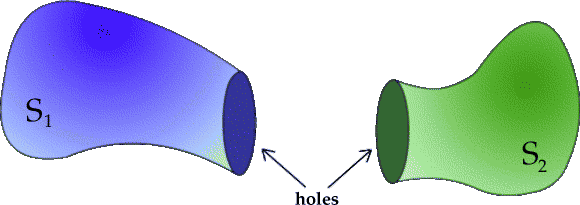Now triangulate both surfaces in such a way that there are exactly $k$ vertices, and hence $k$ edges, on each of the circular boundaries of the holes. Again by deforming the surfaces we may assume that these $k$ vertices and $k$ edges match up exactly when the two surfaces are brought together.
Suppose that the triangulation of $S_1$ has $T_1$ triangles, $E_1$ edges and $V_1$ vertices, and similarly for $S_2$. When the surfaces have been joined together at the edges of the two circular holes, we will have a triangulation of the new surface, which we call $S$, and this triangulation will have exactly $T_1+T_2$ triangles in it. However, it will not have $E_1+E_2$ edges because each of the $k$ edges on the boundary of one hole will be joined to one of the other edges on the other boundary; thus the new triangulation will have exactly $E_1+E_2-k$ edges and, similarly, $V_1+V_2-k$ vertices. Thus $$\begin{eqnarray} \hbox{Euler-number}(S) &=(T_1+T_2) - (E_1+E_2-k) + (V_1+V_2-k)\\ &=(T_1-E_1+V_1) + (T_2-E_2+V_2)\\ &= \hbox{Euler-number}(S_1) + \hbox{Euler-number}(S_2). \end{eqnarray}$$